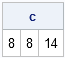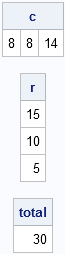The SAS/IML language supports user-defined functions (also called modules). Many SAS/IML programmers know that you can use the RETURN function to return a value from a user-defined function. For example, the following function returns the sum of each column of matrix:

```proc iml; start ColSum(M); return( M[+, ] ); /* sum of all rows, for each column */ finish;   Table = {4 6 5, 3 1 6, 1 1 3}; c = ColSum( Table ); print c;```The RETURN statement only takes a single argument. Nevertheless you can you define a function that returns multiple values. The trick is to return values as output arguments to a user-defined module. Recall that arguments to SAS/IML modules are passed by reference, which means that if a module modifies an argument, the modification is also made to the variables in the "parent environment" at which the module was called. By convention, most SAS/IML programmers use the first few slots of the function's argument list as output parameters, and use the remaining arguments as input parameters.

For example, the following statements define a module that returns three values: the column sum, the row sum, and the total sum of elements in a matrix. Because the module does use the RETURN statement to return a value, use the RUN statement to execute the function, as follows:

```proc iml; /* Return 3 values:|---Output Parameters---|-Input Param-| */ start MarginalSums(ColSum, RowSum, TotalSum, M); ColSum = M[+, ]; /* sum of all rows, for each column */ RowSum = M[ ,+]; /* sum of all columns, for each row */ TotalSum = M[+]; /* sum of all elements */ finish;   run MarginalSums(c, r, total, Table ); /* return three values */ print c, r, total;```The function returns three values with various dimensions. Notice that it is not necessary to pre-allocate the c, r, and total variables. These variables are passed by reference into the module, where they are given the local names ColSum, RowSum, and TotalSum. These variables are assigned values within the module. Upon return, the c, r, and total variables contain the same values, as shown in the printed output.

ShareDistinguished Researcher in Computational Statistics

Rick Wicklin, PhD, is a distinguished researcher in computational statistics at SAS and is a principal developer of SAS/IML software. His areas of expertise include computational statistics, simulation, statistical graphics, and modern methods in statistical data analysis. Rick is author of the books Statistical Programming with SAS/IML Software and Simulating Data with SAS.

1.Ross Bettinger on

How can I teach SAS/IML to return multiple arguments a la MATLAB:

[ ColSum, RowSum, TotalSum ] = MarginalSums( M ) ;

Can I put the results into a character vector and then use indirect address to access the values?

•Try it out and let me know whether you are successful. I have my doubts, but I've never tried it. In my opinion, there is no substantial difference between the two syntaxes: One is a functional syntax, the other is procedural.

2.Ross Bettinger on

Here is the code describing what I want to do:

proc iml;

Table = {8 1 6,
3 5 7,
4 9 2};

start MagicSquare( M ) ;
colSum = M[ +, ] ;
rowSum = M[ , +] ;
totSum = M[ + ] ;

return( 'colSum, rowSum, totSum' ) ;
finish ;

ans = MagicSquare( Table ) ;
print ans ;

print 'scan(ans,1)=' (scan(ans,1)) ;

v1 = value( scan( ans, 1 )) ; v2 = value( scan( ans, 2 )) ; v3 = value( scan( ans, 3 )) ;

quit ;

It fails because the variables are not defined outside of the local scope of the module. Is there any nice way around this? I can create a vector descriptor containing ( # of dimensions, list of dimensions, list of values ) for each variable, and iteratively process each sublist of a list of vector descriptors, but this is inelegant, IMHO. Is there a better way?

•global(colSum, rowSum, totSum)
to the START statement and your example will work.

•Ross Bettinger on

I am familiar with creating a global variable that will be available in the workspace. I am trying to avoid the syntactic requirement of declaring variables to be global before referencing their contents by the artifice of returning a character vector containing their names, but i cannot work around the dynamic scoping with which IML bounds access to variables declared within the body of a module.

•Use the technique that I describe in this article. It is completely equivalent to what you want.

3.If I am running a nonlinear optimization model and I am using a NLPQN function. In addition to I want to the result for more than one variable, 4 of them are defined at the optimization function, and also these 4 variables are defined at the constraints as well as an additional variable defined at the constraints. I compressed all these variables at one vector and I called them at the NLPQN statement but that didn't work, so any suggested ideas that can help?

4.What if I need to create many variables in the module that I don't want to get returned? This strategy won't work, right? So say I write a module that take 3 inputs, generates 10 variables, and I want to return 2 of them. Will this approach work?

•Yes. Let's say A and B are the two variables that you want to return. Define the module by
start MyMod(A, B, x1, x2, x3);
...
finish;

The other eight variables that you define in the module are LOCAL variables. They exist only within the module and vanish when the module returns.

•